Sub­sec­tions

### A.6 Con­stant spher­i­cal po­ten­tials

This ad­den­dum de­scribes the so­lu­tions of the Hamil­ton­ian eigen­value prob­lem in spher­i­cal co­or­di­nates if the po­ten­tial is con­stant.

These so­lu­tions are im­por­tant for many rea­sons. For ex­am­ple, you might want to cre­ate a sim­pli­fied model for the hy­dro­gen atom that way. To do so, you could, for ex­am­ple, as­sume that the po­ten­tial en­ergy has a con­stant neg­a­tive value up to say the Bohr ra­dius and is zero be­yond it. That is not re­ally a very good model for the hy­dro­gen atom. How­ever, it works much bet­ter for nu­cle­ons in atomic nu­clei, chap­ter 14.12.

The so­lu­tions in this note are also im­por­tant for de­scrib­ing ex­per­i­ments in which par­ti­cles are scat­tered from some tar­get, {A.30}. And more fun­da­men­tally, they give the en­ergy states of def­i­nite an­gu­lar mo­men­tum for par­ti­cles in empty space.

#### A.6.1 The eigen­value prob­lem

The Hamil­ton­ian eigen­value prob­lem is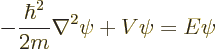In this note it is as­sumed that the po­ten­tialis a con­stant in the ra­dial re­gion of in­ter­est.

To clean the prob­lem up a bit, take the po­ten­tial en­ergy term to the other side, and also the co­ef­fi­cient of the Lapla­cian. That pro­duces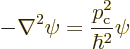where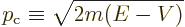The con­stant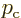is what clas­si­cal physics would take to be the lin­ear mo­men­tum of a par­ti­cle with to­tal en­ergy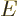and po­ten­tial en­ergy.

#### A.6.2 The eigen­func­tions

Be­cause the po­ten­tial is spher­i­cally sym­met­ric like for the hy­dro­gen atom, the eigen­func­tions are of sim­i­lar form: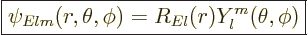(A.17)

Here the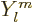are again the spher­i­cal har­mon­ics. These eigen­func­tions have def­i­nite square an­gu­lar mo­men­tum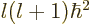where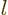is an non­neg­a­tive in­te­ger. They also have def­i­nite an­gu­lar mo­men­tum in the cho­sen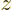-​di­rec­tion equal to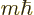, where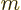is an in­te­ger that sat­is­fies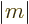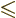.

The ra­dial func­tions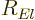in the eigen­func­tions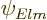are dif­fer­ent from those of the hy­dro­gen atom. De­pend­ing on what­ever is eas­i­est in a given ap­pli­ca­tion, they can be writ­ten in two ways, {D.16}.

The first way is as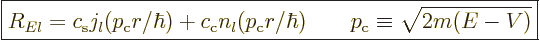(A.18)

Here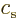and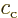are ar­bi­trary con­stants. The func­tions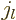and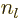are called the “spher­i­cal Bessel func­tions” of the first and sec­ond kinds. Theare also called the “Neu­mann func­tions” and might in­stead be in­di­cated by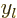or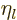.

Ex­pres­sions for these Bessel func­tions can be found in ad­vanced math­e­mat­i­cal hand­books, :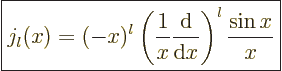(A.19)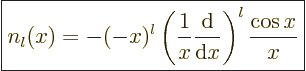(A.20)

The spher­i­cal Bessel func­tions are of­ten con­ve­nient in a re­gion of con­stant po­ten­tial that in­cludes the ori­gin, be­cause the Bessel func­tions of the first kindgive the so­lu­tions that are fi­nite at the ori­gin. (To see that, note that the Tay­lor se­ries of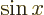di­vided by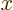is a power se­ries in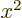, and that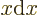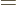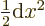.) In par­tic­u­lar for small:(A.21)

Here !! is one of these un­for­tu­nate no­ta­tions. The sec­ond ! means that all even fac­tors are dropped from the fac­to­r­ial.

The Bessel func­tions of the sec­ond kind are sin­gu­lar at the ori­gin and nor­mally do not ap­pear if the ori­gin is part of the con­sid­ered re­gion.

Also, the spher­i­cal Bessel func­tions are real for real. How­ever, in a re­gion where the po­ten­tialis larger than the en­ergyof the par­ti­cles, the ar­gu­ment of the Bessel func­tions in (A.18) will be imag­i­nary.

The other way to write the ra­dial func­tions is as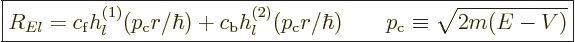where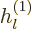and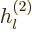are called the “spher­i­cal Han­kel func­tions.”

The spher­i­cal Han­kel func­tions can again be found in ad­vanced math­e­mat­i­cal hand­books, :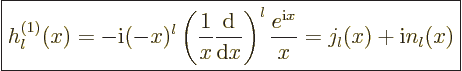(A.22)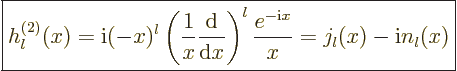(A.23)

The given ex­pres­sions in terms of the spher­i­cal Bessel func­tions are read­ily in­verted to give the Bessel func­tions in terms of the Han­kel func­tions,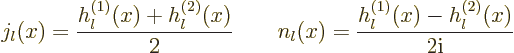(A.24)

For largethe spher­i­cal Han­kel func­tions can be ap­prox­i­mated as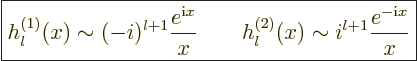(A.25)

This as­ymp­totic be­hav­ior tends to make the Han­kel func­tions more con­ve­nient far from the ori­gin. Ex­po­nen­tials are math­e­mat­i­cally sim­pler and more fun­da­men­tal than the sines and cosines in the as­ymp­totic be­hav­ior of the Bessel func­tions.

#### A.6.3 About free space so­lu­tions

The most im­por­tant case for which the en­ergy eigen­func­tions of the pre­vi­ous sub­sec­tion ap­ply is for par­ti­cles in empty space. They de­scribe en­ergy states with def­i­nite square andan­gu­lar mo­men­tum. How­ever, some­times par­ti­cles in empty space are bet­ter de­scribed by states of def­i­nite lin­ear mo­men­tum. And in some cases, like in scat­ter­ing prob­lems, you need both types of so­lu­tion. Then you also need to con­vert be­tween them.

The en­ergy states in empty space with def­i­nite square andan­gu­lar mo­men­tum are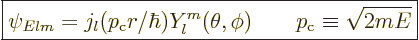(A.26)

These states have square an­gu­lar mo­men­tumand an­gu­lar mo­men­tum in the cho­sen-​di­rec­tion. They are non­sin­gu­lar at the ori­gin.

A state that has def­i­nite lin­ear mo­men­tumpurely in the-​di­rec­tion has an en­ergy eigen­func­tion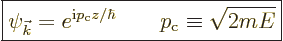(A.27)

This eigen­func­tion is not nor­mal­ized, and can­not be nor­mal­ized. How­ever, nei­ther can the eigen­func­tionabove be. It is the curse of eigen­func­tions in in­fi­nite empty space. An in­tro­duc­tion to the ad­just­ments that must be made to deal with this is given in chap­ter 7.9.

It is some­times nec­es­sary to write a lin­ear mo­men­tum eigen­func­tion of the form (A.27) in terms of an­gu­lar mo­men­tum ones of the form (A.26). Rayleigh worked out the cor­rect com­bi­na­tion a very long time ago, {D.16}: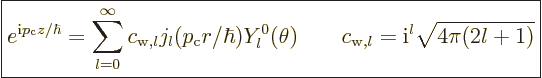(A.28)

Note that only eigen­func­tions with0 are needed.